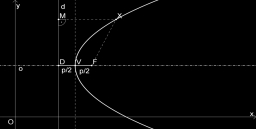# Circumference 26651

A rectangle with sides of lengths a, b (cm) has a circumference of 100 cm. The dependence of its area P (in cm2) on the number a can be expressed by the quadratic function P = sa + ta2. Find the coefficients s, t.

s =  50
t =  -1

### Step-by-step explanation:Did you find an error or inaccuracy? Feel free to write us. Thank you!

Tips for related online calculators
Are you looking for help with calculating roots of a quadratic equation?

#### Grade of the word problem:

We encourage you to watch this tutorial video on this math problem: